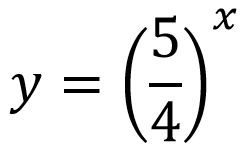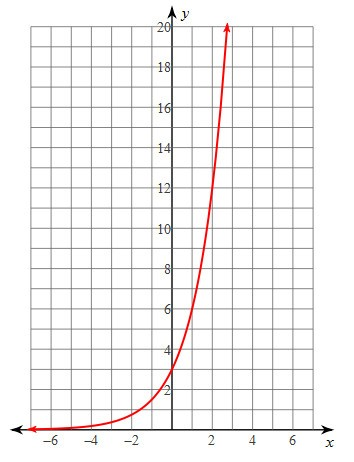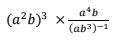# Exponents and Exponential Functions

This set of MCQs helps you brush up on important math topics and prepare you to dive into skill practice.

Start Quiz

What is the balance of \$6000 compounded annually at a rate of 4% for 10 years?

\$8,881.47

\$8,800.47

\$8,801.47

\$8,881.67

Is this exponential growth or decay?Growth

Decay

If f(x) = 2x-9, what is f(3)?

-3

6

3

-6

What is the y-intercept of the function?2

-3

3

-2

The size of a virus is 400 nanometers. 1 nanometer is 1 x 10-9 m. How many viruses can you fit into 1km?

25 x 10^11

2.5 x 10^11

2.345 x 10^11

2.5 x 10^12

A cube has a length of x cm. If the area of the front face of the cube is 64cm^2, what is the value of x?

2

16

8

4

Simplify the following, write the final answer with positive exponents(a)^7

(ab)^5

(ab)^7

(b)^7

Quiz/Test Summary
Title: Exponents and Exponential Functions
Questions: 7
Contributed by: